# What's the underlying frame of the Einstein's Field Equation?

• I
Pyter
My main problems boil down to these:

1) how can you build the stress-energy tensor if you don't have a complete Riemannian manifold yet?
2) If you have a complete Riemannian manifold, you don't need to solve the EFE because you can compute the metric tensor directly from the manifold chart(s).

I'll try to explain it in other words.
(In my previous posts, "map" should be read as "charts", which is the proper terminology).

In relativity, our continuum is modeled after a 4D Riemannian manifold (with Minkowskian metric). The latter is - simplifying - a N-dimensional surface, immersed in a N+1 dimension space, equipped with one or more invertible charts which map a point on the surface to a linear N-space (not necessarily Cartesian, they could be polar or spherical or cylindrical coordinates, but they are linear) and back.

In SR, there are no gravity sources and the motion is inertial, so the chart coincides with the identity chart. We know that ##g_{\mu \nu} = \eta_{\mu\nu}## everywhere.

So far so good.

In GR, instead, the charts depend on the gravity sources (or, equivalently, the accelerated/rotatory motion of the observer).

To determine the position of a point in the manifold our continuum is modeled with, you need its charts, just like when you want to determine the position of a point on a 2D spherical surface with fixed radius, you may use the linear coordinates ##\theta, \phi## and the sine and cosine charts mapping the linear coordinates to the 3D coordinates of the surface points.

To build the SET, you need to specify the position and times of the masses (or of a single extended mass, like a star) in the 4D manifold, and in order to do so you need its charts.
But those charts depends on the manifold's curvature and you don't know it because you haven't solved the EFE yet.
But you can't solve the EFE until you build the SET.

And if you somehow find the right charts to build the SET, why should you need to solve EFE, since you can express the metric tensor analytically by taking the partial derivatives of the chart functions?

And even if you solve the EFE for the ##g_{\mu\nu}## (as a function of the linear coordinates), is it mathematically guaranteed that you can univocally find a chart expressing that metric tensor?

Can you see my conundrum?

Last edited:
Gold Member
In relativity, our continuum is modeled after a 4D Riemannian manifold (with Minkowskian metric). The latter is - simplifying - a N-dimensional surface, immersed in a N+1 dimension space, equipped with one or more invertible charts which map a point on the surface to a linear N-space (not necessarily Cartesian, they could be polar or spherical or cylindrical coordinates, but they are linear) and back.
I think the words I have highlighted in bold might be where you are going wrong. The point about differential geometry is that you don't immerse (or embed) your 4D manifold into any 5D (or larger) space. I suspect you are thinking that you need to find the embedding map that maps 5D coordinates into 4D coordinates. You don't. You just have 4D coordinates and you need to find the manifold's metric expressed in terms of those coordinates. That's it, there is nothing more to do.

•Klystron and cianfa72
Mentor
1) how can you build the stress-energy tensor if you don't have a complete Riemannian manifold yet?
Often we know the distribution of matter and energy so we know the stress-energy tensor. The derivation of the Schwarzschild metric is an example: the SE tensor is zero everywhere. A more complex example with a known but non-zero SE tensor would be the Schwarzschild interior solution. We solve the EFEto find the metric that is consistent with the known SE tensor and the symmetries and boundary conditions of our problem.
In relativity, our continuum is modeled after a 4D pseudo-Riemannian manifold (with locally Minkowskian metric). The latter is - simplifying - a N-dimensional surface, immersed in a N+1 dimension space
Bold text above has been added are added for the sake of precision, ignore if you agree. More important: that bit about embedding in an N+1 dimensional space is incorrect. GR has no notion of embedding four-dimensional spacetime in five-dimensional space; everything is described in terms of points and curves in the four-dimensional manifold. Knowing the metric allows us to calculate the geometrical relationships between these points and curves without introducing a higher-dimensional space.

There is an analogy with navigating around on the curved two-dimensional surface of the earth. As long as we’re only moving in two dimensions we work with latitude and longitude and the metric relationships (ship captains call these relationships “spherical trigonometry” but they’re a specific example of the general concept of the metric at work) between these coordinates. The cartesian x,y,z coordinates of the three-dimensional space around the Earth are completely unhelpful - we don’t need them, we don’t calculate them, we don’t think about them.
In SR… we know that ##g_{\mu \nu} = \eta_{\mu\nu}## everywhere.
Another small point, you can ignore if you agree: that statement is only true if we choose to use Minkowski coordinates. Use, for example, Rindler or spherical coordinates and the components of ##g## will take on different values. But it’s the same flat spacetime and the same (trivial) solution of the EFE.
But those charts depends on the manifold's curvature and you don't know it because you haven't solved the EFE yet.
But you can't solve the EFE until you build the SET.
You do have your ansatz, and you can match it to your boundary conditions - and as I said above you often do know the SE tensor.

You are right that there is no general recipe for finding the metric for an arbitrary configuration of stress-energy. As with most problems involving complex differential equations, we need an inspired guess as to the form of the solution before we can make progress. It’s worth looking at the derivations of some of the exact solutions of the EFE to see what techniques are useful.

Last edited:
Mentor
What I know about curvilinear coordinates system, i.e. to make a concrete example locating points on the surface of a 3D sphere (that is a 2D curved surface immersed in a 3D space), is that you map them to Cartesian coordinates.
In some cases you can, but there is no requirement that you must always do so or even that you must always be able to do so. Since your arguments appear to hinge on the assumption that you must always do this, your arguments are based on a false premise.

Mentor
1) how can you build the stress-energy tensor if you don't have a complete Riemannian manifold yet?
The EFE is a differential equation. You can solve a differential equation in a local neighborhood of some point without having to know the full manifold. In fact, in many cases, including famous solutions of the EFE such as Schwarzschild, it took decades from the initial discovery of the solution before the full maximal analytic extension of the manifold was known.

If you have a complete Riemannian manifold, you don't need to solve the EFE because you can compute the metric tensor directly from the manifold chart(s).
That's true, but you also have no guarantee that when you compute the Einstein tensor of this known metric and use the EFE to see what it implies about the stress-energy tensor, that you will get anything that is physically reasonable. That's why physicists work the other way: they start with some physically reasonable stress-energy tensor and solve the EFE to see what metric it implies.

To build the SET, you need to specify the position and times of the masses (or of a single extended mass, like a star) in the 4D manifold, and in order to do so you need its charts.
You need some chart. There are an infinite number of possible charts on any manifold. If you know that the manifold has some symmetries, you can pick charts that match up with those symmetries (for example, the standard Schwarzschild-type chart for spherical symmetry), but that still leaves quite a bit of freedom of choice of chart.

But those charts depends on the manifold's curvature
No, they don't. The metric in a given chart depends on the manifold's geometry, but knowing a chart is not the same as knowing the full metric. You might be able to write down an expression for the metric that has fewer than ten unknown functions in it (ten is the most general number possible since the metric is a symmetric 2nd-rank tensor), and/or with functions of fewer than all four coordinates.

For example, if you write the most general metric for a spherically symmetric spacetime in the standard Schwarzschild chart, your metric will have two unknown functions in it, functions of only the radial and time coordinates, which you then have to determine by solving the EFE, which in turn means you have to make some assumption about the stress-energy tensor. You can do all that without knowing the full metric.

••Klystron, cianfa72 and vanhees71
cianfa72
You need some chart. There are an infinite number of possible charts on any manifold. If you know that the manifold has some symmetries, you can pick charts that match up with those symmetries (for example, the standard Schwarzschild-type chart for spherical symmetry), but that still leaves quite a bit of freedom of choice of chart.
So, for example, I believe you can solve EFE for the orbits of solar system's planets starting from Schwarzschild spacetime in Schwarzschild chart with origin (coordinate radius ##r=0##) in the Sun.

Pyter
I think the words I have highlighted in bold might be where you are going wrong. The point about differential geometry is that you don't immerse (or embed) your 4D manifold into any 5D (or larger) space. I suspect you are thinking that you need to find the embedding map that maps 5D coordinates into 4D coordinates. You don't. You just have 4D coordinates and you need to find the manifold's metric expressed in terms of those coordinates. That's it, there is nothing more to do.
So far I've only seen examples of charts mapping from a N+1-dimension Euclidean space to a N-dimension space. Anyway you're right, the main purpose of the metric tensor is to get rid of the N+1-dimension (so-called ambient) space and to work only with N coordinates.

My point is that to map your manifold to the linear space you either need the charts (which give you a superior knowledge over the metric tensor, since you can obtain its components by taking the partial derivatives with respect to the linear coordinates and doing scalar products of the resulting vectors), or the metric tensor. Before solving the EFE you don't have any of them, and to solve the EFE you need to build a SET, function of the linear coordinates, for which you need either the charts or the metric tensor.
A more complex example with a known but non-zero SE tensor would be the Schwarzschild interior solution. We solve the EFEto find the metric that is consistent with the known SE tensor
This only shifts the problem from a discrete number of points with finite mass to an infinite number of points with infinitesimal mass (the interior of the fluid). You need to specify their linear coordinates in the SET anyway.

that bit about embedding in an N+1 dimensional space is incorrect. GR has no notion of embedding four-dimensional spacetime in five-dimensional space; everything is described in terms of points and curves in the four-dimensional manifold. Knowing the metric allows us to calculate the geometrical relationships between these points and curves without introducing a higher-dimensional space.

As long as we’re only moving in two dimensions we work with latitude and longitude and the metric relationships (ship captains call these relationships “spherical trigonometry” but they’re a specific example of the general concept of the metric at work) between these coordinates. The cartesian x,y,z coordinates of the three-dimensional space around the Earth are completely unhelpful - we don’t need them, we don’t calculate them, we don’t think about them.
If that's true, from the procedural standpoint, how would you find the latitude and longitude of two points on the Earth's surface without leaving it, using only curved rulers or light/radio waves running along its surface?
In some cases you can, but there is no requirement that you must always do so or even that you must always be able to do so.
If you want to express the SET as function of the linear coordinates, you need either a metric or - even better - the charts.
The EFE is a differential equation. You can solve a differential equation in a local neighborhood of some point without having to know the full manifold.
Since you cite a local neighborhood, I guess you mean to solve the EFE under the initial assumption that the identity chart applies? This would conflict with the requirement that the solution must yield a non-flat space, rendering the identity chart invalid.
You need some chart.
Yes, but at least one.
No, they don't. The metric in a given chart depends on the manifold's geometry, but knowing a chart is not the same as knowing the full metric.
The charts also contain info on the manifold's curvature and more complete, since you can derive the metric tensor from the charts. Or at least you can derive the metric in the part of the manifold covered by that particular chart, if that's what you meant by "knowing the full metric".

Mentor
So, for example, I believe you can solve EFE for the orbits of solar system's planets starting from Schwarzschild spacetime in Schwarzschild chart with origin (coordinate radius ##r=0##) in the Sun.
This isn't solving the EFE. You already have the solution: Schwarzschild spacetime (more precisely, Schwarzschild spacetime for the vacuum region outside the sun). All you're doing by solving for planetary orbits is solving the geodesic equation for that known solution of the EFE.

Note, btw, that our Solar System measurements are accurate enough that we can spot inaccuracies in the scheme just described, due to the fact that the planets are not test particles but are significant sources of gravity on their own. The approximation scheme used to address this works by ignoring non-linearities and solving for the motion of each planet by simply adding the contributions to the spacetime geometry from the Sun and all the other planets. But none of this is solving the EFE; it's just solving the geodesic equation.

•cianfa72 and PeroK
Mentor
So far I've only seen examples of charts mapping from a N+1-dimension Euclidean space to a N-dimension space.
Then you need to spend some time with some GR textbooks, working through actual examples from GR, not just using your intuition developed from other fields.

to map your manifold to the linear space
Which, as you have already been told, you never need to do to solve the EFE. You simply do not understand how the EFE is actually solved. You need to spend some time with some GR textbooks, learning how the EFE is actually solved by working through actual examples.

You need to specify their linear coordinates in the SET anyway.
No, you don't. You can specify the SET in any coordinate chart you like.

If you want to express the SET as function of the linear coordinates, you need either a metric or - even better - the charts.
You need a chart, but, as I have already said, the metric expressed in this chart can and will have unknown functions of the coordinates in it. Solving the EFE means solving for these unknown functions.

You keep making assertions that are simply wrong, I suspect because you have simply not spent any time actually looking at a GR textbook and seeing how actual solutions to the EFE work in GR. And you are continuing to make the same wrong assertions even though you have been corrected multiple times. There is no point in continuing to go around in circles.

Since you cite a local neighborhood, I guess you mean to solve the EFE under the initial assumption that the identity chart applies?
No. A GR textbook will explain what I meant. This is a very basic concept.

The charts also contain info on the manifold's curvature
No, they don't. The metric contains information on curvature, once all unknown functions in the metric have been solved for. But you don't need to have done all that to have a chart. Once again, you continue to make these wrong assertions even though you have been corrected multiple times already. There is no point in continuing to go around in circles.

Mentor
how would you find the latitude and longitude of two points on the Earth's surface without leaving it, using only curved rulers or light/radio waves running along its surface?
You wouldn't. Latitude and longitude are global coordinates, not local ones. You can't find them by purely local measurements.

If you wanted to find the metric of the Earth's surface based on purely local measurements within the surface, here is how you would proceed, by analogy with how the corresponding task would be done in GR:

(1) Set up local coordinates any way you want. The usual method would be to start by labeling the point where you are as ##(0, 0)##, and then marking out two perpendicular straight lines through that point and labeling them as your two different coordinate axes. Strictly speaking, there is no requirement for the coordinate axes to be perpendicular, only linearly independent; but making them perpendicular, at least locally, makes the calculations easier when you want to compute the curvature.

(2) Pick one coordinate axis, call it ##a## (I'll avoid using the standard Cartesian names since we will be finding that the manifold in question is not flat), and mark off equal increments of some convenient unit distance (such as the length of your meter stick) along it from ##(0, 0)##. Then mark out perpendicular straight lines crossing the axis at each of your marked points. At each of the crossing points, these perpendicular straight lines will be tangent to the coordinate grid lines for your other coordinate, call it ##b##.

(3) Extend each of the perpendicular straight lines by purely local means--i.e., just looking at that particular line and keeping it straight by extending it in the same direction the way a surveyor would, without regard to its relationship with any neighboring lines--and see if they converge or diverge. If they do, the manifold is curved, at least in that local region. If they don't, the manifold is flat, at least in that local region.

On Earth, you will find that the lines converge (globally, what you are doing is drawing segments of adjacent great circles that all start off crossing another great circle at right angles, and those will of course converge), indicating that the manifold is curved. By measuring the rate of convergence, you can find the numerical value of the curvature (which, since this is a 2-dimensional manifold, will just be a single number; to put it another way, the Riemann tensor of this manifold has only one component).

In terms of your local coordinate chart, you will find that the perpendicular straight lines you have drawn will not be identical with the coordinate grid lines of ##b##. In terms of the metric written in this coordinate chart, you will find that it looks exactly Euclidean at point ##(0, 0)##, but picks up non-Euclidean terms as you move away from ##(0, 0)##. (Obviously, then, these coordinates are not latitude and longitude, as I said above.)

The method I have described above is analogous to setting up what are called Riemann normal coordinates in GR, centered on a chosen point of spacetime. These coordinates are chosen to "look Minkowskian" at the chosen point, just as the coordinates I described above "look Euclidean" at the chosen point. Most GR textbooks discuss this at a fairly early point in their exposition.

•PeroK
Pyter
Thinking about what was said in this thread, I've found a way out of my impasse.

I considered the RHS/SET in the EFE as the "independent variables" in the equations, and the LHS/Einstein's tensor as the "dependent variables", thinking it was the only side containing ##g_{\mu\nu}##.
So I thought that you needed to fully specify the SET in the spacetime region under observation before solving the equation. But that would be tantamount to completely express (by observation) the equations of motions of the energy/matter/fields between the time boundaries of the region, which would render the solution of the EFE useless.

Now I'm aware that both sides of the EFE contribute to ten differential equations whose unkown are the components of ##g_{\mu\nu}##, and thus also the RHS depends on the linear coordinates through the unknown functions ##g_{\mu\nu}##.

The physical observation of your masses only set the boundary conditions of the EFE, and they contain the unknown ##g_{\mu\nu}## in a parametrized form, for instance in the measurement of your distance from objects of known mass.

Am I on the right track?

Pyter
You wouldn't. Latitude and longitude are global coordinates, not local ones. You can't find them by purely local measurements.
You could, but only if you had the charts or the metric tensor on the whole manifold. This way, knowing the length of your measured paths of light from/to the objects, and knowing that they move along a geodesic, you could project these paths on the linear (coordinate) space and find the coordinates of every point.

Mentor
Am I on the right track?
@PeterDonis and I have both suggested that you look at the derivation of some of the known solutions to the EFE. Working through a few of these will tell you more about how these problems are solved than any amount of general principles.

Pyter
So far I've only seen examples of charts mapping from a N+1-dimension Euclidean space to a N-dimension space.
Then you need to spend some time with some GR textbooks, working through actual examples from GR, not just using your intuition developed from other fields.
I think I've spent on them quite some time already :). And I've found those charts in those textbooks. What would be another example of a legit chart on a manifold that doesn't map a N+1 space into a N space? Charts for a 2D spherical surface, for instance.
@PeterDonis and I have both suggested that you look at the derivation of some of the known solutions to the EFE. Working through a few of these will tell you more about how these problems are solved than any amount of general principles.
So far I've looked into the most famous one, the Schwartzschild solution, but that didn't answer my questions on the general case.

cianfa72
This isn't solving the EFE. You already have the solution: Schwarzschild spacetime (more precisely, Schwarzschild spacetime for the vacuum region outside the sun). All you're doing by solving for planetary orbits is solving the geodesic equation for that known solution of the EFE.
Yes definitely, my terms were misleading. So we actually solve the geodesic equation for planet orbit in Schwarzschild coordinates ##(r,\theta,\phi,t)##. From the solution then we can calculate for example the proper time needed to complete an orbit around the Sun.

Mentor
Am I on the right track?
No.

I think I've spent on them quite some time already :).
It doesn't seem like it from what you're posting.

I've found those charts in those textbooks.
What textbooks?

What would be another example of a legit chart on a manifold that doesn't map a N+1 space into a N space? Charts for a 2D spherical surface, for instance.
Latitude and longitude on a sphere. These are coordinates just on the sphere, and do not either account for or require the sphere to be embedded in a higher dimensional space.

The same is true of every chart used in GR.

So far I've looked into the most famous one, the Schwartzschild solution
It doesn't seem like it, since none of the descriptions you have given of how you think the EFE is solved bear any resemblance at all to how the EFE is actually solved to obtain the Schwarzschild solution.

that didn't answer my questions on the general case.
If your understanding of that one special case is wrong, as it seems to me to be, of course you're not going to learn anything about the general case from it.

•vanhees71
2022 Award
I wrote an Insight on solving the EFEs for a fairly simple non-vacuum case, here, which may be of interest. It's a fairly bull-at-a-gate approach, but there are ways to make it more elegant discussed at the end.

•vanhees71 and Pyter
Pyter
No.
Closer at least?
What textbooks?
For instance, in Intro to Differential Geometry and General Relativity - S. Waner, page 10, I've found: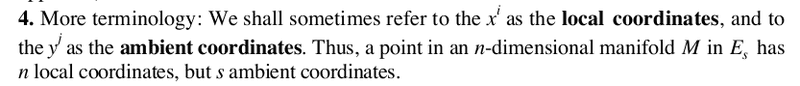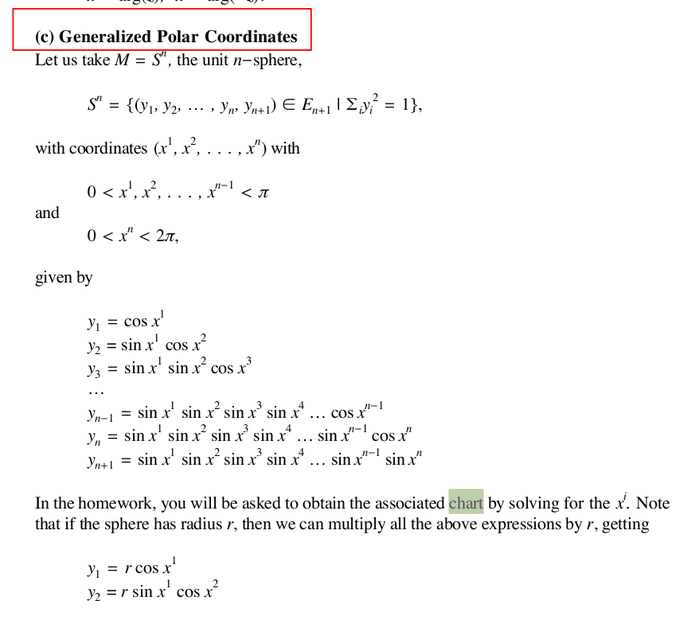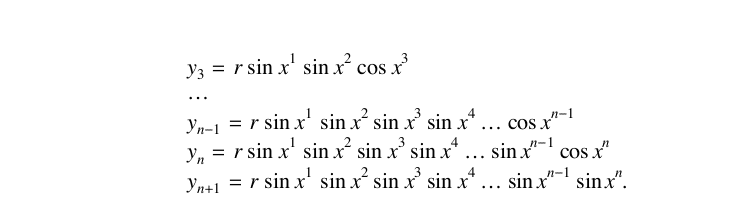Latitude and longitude on a sphere. These are coordinates just on the sphere, and do not either account for or require the sphere to be embedded in a higher dimensional space.

The same is true of every chart used in GR.
See above. I count n+1 dimensions for the ambient space, n dimensions for the sphere.
If your understanding of that one special case is wrong, as it seems to me to be, of course you're not going to learn anything about the general case from it.
That's why I'm on this forum asking you GR megaexperts for help :)

Mentor
Closer at least?
No.

For instance, in Intro to Differential Geometry and General Relativity - S. Waner, page 10, I've found:
Hm. Is this the only GR textbook you've looked at? Its approach is completely different from that of any GR textbook I've read. Part of the problem may be that it's written by a mathematician, not a physicist; but it's still surprising to me because a mathematician should understand even better than a physicist the theory of the intrinsic curvature of manifolds, without regard to the usage or even the existence of an embedding in a higher dimensional space.

In short, I do not think this is a good reference.

That's why I'm on this forum asking you GR megaexperts for help :)
But you're not listening to the help we're giving you. You keep making the same wrong assertions even after you've been told they're wrong multiple times. If you want help, you have to listen to it.

For some specific examples, you can look at the Insights articles that @Ibix and I linked to. Also here are some examples from GR textbooks and lecture notes:

Sean Carroll's lecture notes on GR , Chapter 7, discusses the Schwarzschild solution and how it can be obtained. (Carroll also has a textbook which contains all the material in the lecture notes plus more, but the notes are nice because they're available for free online.)

Carroll's notes, Chapter 8, discusses the FRW solutions used in cosmology and how they can be obtained.

Misner, Thorne, & Wheeler, Chapter 23, discusses how to construct solutions describing static, spherically symmetric stars.

MTW, Chapter 27, discusses how to construct idealized cosmological models of the universe.

MTW, Chapters 31 and 32, discuss black hole solutions and various coordinate charts used for them.

Wald, Chapter 5, discusses cosmological models and solutions.

Wald, Chapter 6, discusses the Schwarzschild solution and its derivation.

 https://arxiv.org/abs/gr-qc/9712019

•vanhees71 and dextercioby
Pyter
The Schwartschild solution in the textbook I've just mentioned is built to find g** of the form: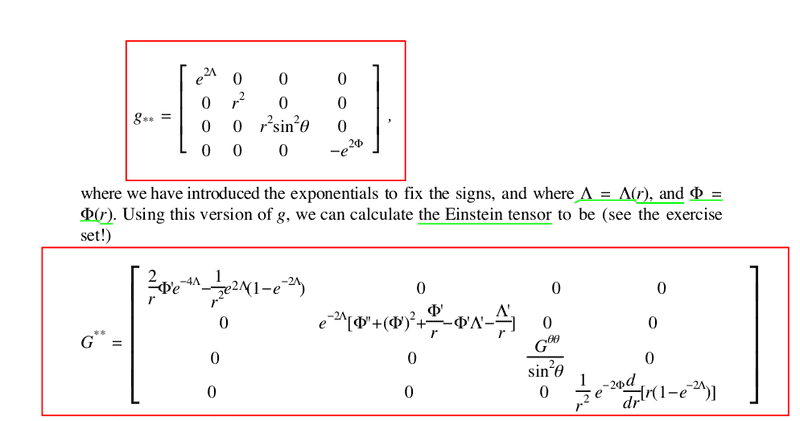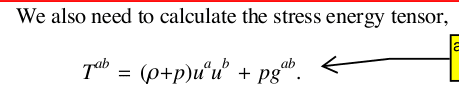(So I was right, the SET is also function of g**).
After setting some more constraints, T** becomes: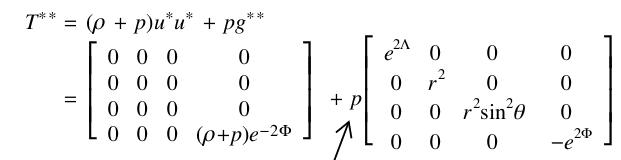Then, after imposing that the equations of motions: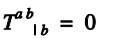are satisfied, we have the additional constraint: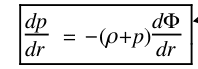Then it goes on to solve the differential equations for the single components, finding: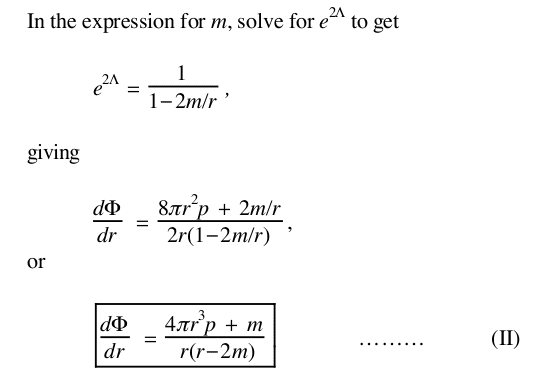Just looking at this, you'd think that the r in the equations is the actual radius of the star measured in our continuum, while it's a linear (local) coordinate, and that enough is a source of confusion to me.
Also the variable t is a linear/local coordinate, which doesn't match the actual time measured by an external observer. You compute the latter through the metric.

#### Attachments

Pyter
Besides, I really don't know how I could infer the general case from that special case.
a mathematician should understand even better than a physicist the theory of the intrinsic curvature of manifolds, without regard to the usage or even the existence of an embedding in a higher dimensional space.
Intuitively I thought that the very concept of "curvature" implied the embedding on a higher space.
If you only have a 1D space, you wouldn't have "curved" or "straight" lines, you'd only have "lines", because the curvature only appears in a 2D space or higher. Same thing for the 2D, and higher dimensions, surfaces.
After all, the curvature of a surface is the rate of change of its normal vector, and that is "outside" the surface, in a higher dimension space.

Mentor
The Schwartschild solution in the textbook I've just mentioned is built to find g** of the form:
Yes, that's one of the possible ways of writing the metric for a spherically symmetric spacetime. Note that it has two unknown functions in it, ##\Lambda## and ##\Phi##.

(So I was right, the SET is also function of g**).
That SET is the general form of the SET for a perfect fluid. In order to see why the metric appears in it, write it this way:

$$T^{ab} = \rho u^a u^b + p \left( g^{ab} + u^a u^b \right)$$

The tensor multiplying ##p## is the projection tensor into spacelike hypersurfaces orthogonal to the fluid. In other words, the metric appears in it in order to reflect the physical fact that, in the rest frame of the fluid, the pressure is purely spatial. (Note that this expression is valid in any coordinate chart.)

Just looking at this, you'd think that the r in the equations is the actual radius of the star measured in our continuum
No, you wouldn't. Look at the metric. The increment of radial distance is not ##dr##, it's ##e^\Lambda dr##. The coordinate ##r## is, again looking at the metric, the "areal radius", i.e., the area of a 2-sphere with radial coordinate ##r## is ##4 \pi r^2##.

while it's a linear (local) coordinate
No, it isn't. It's a global coordinate on the spacetime.

and that enough is a source of confusion to me.
Your confusion appears to be the result of an incorrect understanding of what you are reading. See above.

Also the variable t is a linear/local coordinate
No, it isn't. It's a global coordinate on the spacetime. The chart you are looking at is a global chart, not a local one.

which doesn't match the actual time measured by an external observer.
Yes, it does, for the usual meaning of "external observer". The ##t## coordinate matches proper time for an observer at infinity. It does not match proper time for an observer at finite ##r##; the increment of proper time for such an observer is ##e^\Phi dt##, not ##dt##. But such an observer is a "local" observer, not an "external" observer.

You compute the latter through the metric.
You compute the proper time for an observer at finite ##r## using the metric, yes, as I just did above. (Note, though, that that expression is only valid for an observer who "hovers" at the same ##r##, ##\theta##, ##\phi## forever.)

Mentor
I really don't know how I could infer the general case from that special case.
If you look at the Insights articles or the textbook references that have been given, you will see specific solutions being derived using the same general method.

Intuitively I thought that the very concept of "curvature" implied the embedding on a higher space.
Then you thought wrong. It doesn't. As you have already been told repeatedly in this thread.

If you only have a 1D space, you wouldn't have "curved" or "straight" lines, you'd only have "lines", because the curvature only appears in a 2D space or higher.
That is correct, but it does not generalize the way you claim.

Same thing for the 2D, and higher dimensions, surfaces.
Wrong. For any manifold of 2 or more dimensions, there is a well-defined intrinsic curvature that does not require the usage or even the existence of an embedding in any higher dimensional space.

the curvature of a surface is the rate of change of its normal vector
That is extrinsic curvature, not intrinsic curvature (intrinsic curvature is sometimes also called Gaussian curvature in the literature). The spacetime curvature referred to in GR is intrinsic curvature. If all of your knowledge of curvature up to now has been of extrinsic curvature, no wonder you're having problems. You need to study the definition and examples of intrinsic curvature so that you understand it. It is a different concept from extrinsic curvature.

Pyter
The chart you are looking at is a global chart, not a local one.
I don't understand what you mean by "local" or "global" chart. To me a chart is a set of functions mapping the N+1 coordinates of the ambient (embedding) space to the N local coordinates of the N-dimensional manifold.
Here's another example of that type of charts: http://berkeleyscience.com/gr.htm
Why should you use curvilinear coordinates for T** and G** when you have the linear, local coordinates at your disposal?

Mentor
To me a chart is a set of functions mapping the N+1 coordinates of the ambient (embedding) space to the N local coordinates of the N-dimensional manifold.
That's not what a "chart" is in any GR textbook I've read, certainly not in any of the references you've been given, or in any of the Insights articles you've been referred to, or in the examples you yourself have been posting. In the example you've given, Schwarzschild coordinates in a spherically symmetric spacetime, the spacetime itself is a 4-dimensional manifold and you have 4 coordinates, ##t##, ##r##, ##\theta##, ##\phi##. Each point in the spacetime is labeled by a 4-tuple of coordinates, with appropriate properties of continuity. There is no "mapping" to any embedding space anywhere.

Since the chart covers the entire spacetime (at least in the example you're discussing--note that this would not be true in the case of a black hole, but you should not even try to understand that case until you understand the simpler case of a spherically symmetric massive body surrounded by vacuum, which is what the example you've been posting is describing), it is global. A "local" chart in GR is something like the Riemann normal coordinates I described earlier; the coordinates are "local" because the expression for the metric in these coordinates is exactly valid only at the single chosen point on which the coordinates are centered, and becomes more and more inaccurate as you get further away from that point. The expression for the metric in Schwarzschild coordinates, however, is valid everywhere.

Why should you use curvilinear coordinates for T** and G** when you have the linear, local coordinates at your disposal?
You do not have "linear, local coordinates" at your disposal, not the way you are using that term. You do not have any embedding of spacetime in any higher dimensional Euclidean space. The examples you have given of embeddings are not of spacetime, they're of 2-surfaces in Euclidean 3-space. Those examples do not generalize to spacetime. They just don't. If you continue to insist on treating spacetime this way, this thread will be closed as that is not the way it is done in the GR literature and we cannot help you if you insist on continuing to try to understand it that way. It won't work.

Pyter
If you look at the Insights articles or the textbook references that have been given, you will see specific solutions being derived using the same general method.
It would take me weeks, I hoped to have a quicker answer.
So far what I gleaned from this thread is: you can choose whatever type of coordinates you see fit. Of course the resulting g** will vary accordingly, I might add.
The only thing I'm missing is how to link the physical observation of the mass points to the setup of the EFE in the general case.

Mentor
Here's another example of that type of charts: http://berkeleyscience.com/gr.htm
You seem to have a talent for picking bad sources. I see no point in trying to deconstruct what is wrong with this one when we have given you a number of good sources already.

Pyter
You seem to have a talent for picking bad sources. I see no point in trying to deconstruct what is wrong with this one when we have given you a number of good sources already.
It's based on Richard L. Faber, "Differential Geometry and Relativity Theory", Marcel Dekker, 1983.
I guess also that one goes in the trashcan.

Mentor
It would take me weeks, I hoped to have a quicker answer.
As Euclid is supposed to have said to a king who wanted a quicker answer from him, there is no royal road to learning.

You do realize that those of us experts who are trying to help you here have spent years or decades studying GR, right?

So far what I gleaned from this thread is: you can choose whatever type of coordinates you see fit. Of course the resulting g** will vary accordingly, I might add.
Yes, that is correct.

The only thing I'm missing is how to link the physical observation of the mass points to the setup of the EFE in the general case.
Ok, here's the fully general case. Pick coordinates ##x^\mu##, where ##\mu## ranges from ##0## to ##3##. The general expression for the metric in these coordinates is:

$$ds^2 = g_{\mu \nu} x^\mu x^\nu$$

where ##g_{\mu \nu}## is a symmetric, 2nd-rank tensor with 10 independent components. Each of those 10 independent components is an unknown function of the four coordinates. (I have used the Einstein summation convention in the above, so the expression on the RHS is actually a sum of 10 terms in the general case.)

Now just compute the Einstein tensor of the above and set each of its 10 independent components (which will be a differential equation in the ##g_{\mu \nu}## and their first and second derivatives) equal to the corresponding component of the SET, which will be another function of the four coordinates, which might be known or unknown. (If ##g_{\mu \nu}## appears in the SET, that just becomes part of the unknown function of the four coordinates for that SET component.)

You now have a system of 10 second order differential equations for (possibly, depending on how many SET components you know) up to 20 unknown functions of the four coordinates. Then all you have to do is solve it. Good luck.

Mentor
It's based on Richard L. Faber, "Differential Geometry and Relativity Theory", Marcel Dekker, 1983.
I guess also that one goes in the trashcan.
Are these the only textbooks you have read? They're both by mathematicians. Have you read any textbooks by physicists? Such as, oh, I don't know, the two classic textbooks in the field that I've already referenced (MTW and Wald)? Or the nice introductory lecture notes by Carroll, another physicist?

Mentor
It's based on Richard L. Faber, "Differential Geometry and Relativity Theory", Marcel Dekker, 1983.
I guess also that one goes in the trashcan.
Based on the seriously mistaken understanding these books appear to have given you, yes, they should go in the trash can.

Pyter
Are these the only textbooks you have read? They're both by mathematicians. Have you read any textbooks by physicists? Such as, oh, I don't know, the two classic textbooks in the field that I've already referenced (MTW and Wald)? Or the nice introductory lecture notes by Carroll, another physicist?
As I've answered in another thread, I also read the Dirac textbook, but it's very criptic even if it covers a lot of subjects that others don't, like the EM field component of the SET.
I have the MTW in my library but it's more than 1000 pages thick, I think I'll go with the Wald.
BTW are you little biased towards mathematicians or is it just an impression of mine :)?
Now just compute the Einstein tensor of the above and set each of its 10 independent components (which will be a differential equation in the and their first and second derivatives) equal to the corresponding component of the SET, which will be another function of the four coordinates, which might be known or unknown. (If appears in the SET, that just becomes part of the unknown function of the four coordinates for that SET component.)
Ok but how the (at least initial) observations of the masses enters the picture, as in the solar system scenario I've brought up?

Mentor
You now have a system of 10 second order differential equations for (possibly, depending on how many SET components you know) up to 20 unknown functions of the four coordinates. Then all you have to do is solve it. Good luck.
Of course no physicist actually does this. That's why asking for "the general case" of solving the EFE is pointless.

What physicists actually do is look for various different ways of reducing the number of unknowns in the above scheme. The most obvious way is to assume various symmetries for the spacetime. For example, spherical symmetry, as I said before, reduces the number of unknown functions in the metric to two; and furthermore, even if we make no other assumptions, we know that those two functions can only be functions of the ##r## and ##t## coordinates, not the angular coordinates (assuming we are using standard Schwarzschild coordinates). And it turns out that in this general case there are only three independent components of the EFE, so we have three independent components of the SET that are possibly unknown functions. That reduces us to three differential equations for five unknown functions, so all we have to do is make some specific assumption for two of the unknown functions and we have a well-defined solution. (What I have just said is a quick summary of the Insights article of mine on the Einstein Field Equation for a spherically symmetric spacetime.)

But of course there are many other possible symmetries one could try; or one could try other ways of reducing the number of unknowns, by making other restricting assumptions. So there is not just one "general case" of solving the EFE. There are lots and lots of different special cases, and the best way to understand how they work is to actually go look at them and work with them, one at a time. The references we have already given are a good start.